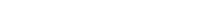# Math riddles Level 67 Answer with Hints and solutionsMath riddles game level 67 Answer with solution android game developed by Black games. Scroll Below to find details.

Math Riddles tests your IQ with mathematical puzzles. Challenge yourself with different levels of math puzzles and stretch the limits of your intelligence. Every IQ game is prepared with an approach of an IQ test. Improve your mathematics, challenge your brain with this game. There are many hard levels which you may not have solved then you can take help from here.

Math app level 67:Solution: the sum of two outer opposite numbers is equal to the number in the inner circle

10+2=12

If there is any Doubt/discrepancy then please let me know in comments or you are unable to understand solution then I will surely help you.

### One thought on “Math Riddles App Level 67 Solution Android”

•May 8, 2019 at 6:59 pm